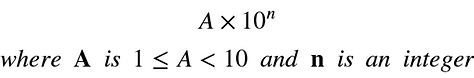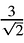top of page## Standard Form

In this section we will quickly go over how numbers are used in practical applications like converting currencies, calculating areas of lands, converting to standard form for doing calculations etc.

Standard form is also referred to as standard index form or scientific notation. Remember in the precious chapter, Units and Accuracy , we discussed about how metric prefixes are used. They are used together with the SI units make it easier to express very large or very small numbers using a single type of unit. Similarly, standard form is also used to make large numbers or small numbers more manageable.

Standard form is written in powers of ten. Therefore, you don't have to use the metric prefixes but instead just use powers of 10 and express the number with the desired number of significant numbers.

The general form of the scientific notation is:Let's say we want to convert 1000 to standard form. Looking at the general form above we have to make sure that A is greater than or equal to 1 but less than ten. For 1000 we should take 1 to be A. For 1 to be 1000, n would have to be 3; therefore, 1000 = 1 x 10^3.

What if the number was 1300. In this case we can't take A to be 13 because it is greater than 10. We will have to take A to be 1.3; therefore, n will have to be 3. So, 1300 = 1.3 x 10^3.

Let's work out a couple more examples:

Example 1

Express the following number in standard form:

a) 185460000

b) 45000000

c) 0.1

d) 0.000234

a) First we have to figure out what A is. We have to change 18546 to A such that the conditions in the rule shown above are met:b) First we have to figure out what A is. 4.5 meets the conditions so we can use that as A:c) Even these numbers are already in decimal we still follow the same process we did for the previous examples. We can't use 0.1 since it is smaller that 1; therefore, we use 1 as A:d) In this case the perfect value for A is 2.34 since it meets the conditions:Example 2

Express the following number in normal form:

a) 1.2 x 10^(-3)

b) 8.99 x 10^5

c) 8.99 x 10^(-5)

d) 1.23 x 10^2

a) 1.2 x 10^(-3) =  1.2 / 1000 = 0.0012

Here we divide by 1000 because 10 is raised to a negative power. If you cant remember how to deal with powers check out the Powers and Roots chapter before proceeding.

b) 8.99 x 10^5 = 8.99 x 100000 = 899,000

c) 8.99 x 10^(-5) = 8.99 / 100000 = 0.0000899

d) 1.23 x 10^2 = 1.23 x 100 = 123

Converting back to ordinary numbers is not hard since all you have to do is either divide or multiply A by the relevant multiple of 10.

## Normal Practical Calculations

In this section we will just look at how to apply what we learned so far in this unit to solve practical problems.

Example 3

Let's assume that you are going abroad for vacation. You have LKR 500,000 with you and you need to convert it to foreign currency. You are going to U.S. The current buying rate for the USD is LKR 182; selling rate is LKR 186. How much USD will you have after exchanging currencies?

This is a pretty straight forward question if you know what the buying rate and selling rate is. These rates are given by the banks. So the buying rate is the rate at which a bank will buy a foreign currency from you. The selling rate is the rate at which the bank will sell a foreign currency to you.

So you have to look at the buying rate when you have a foreign currency and want to exchange it for a local currency. You look at the selling rate when you have local currency with you but instead want a foreign currency.

In this case you want to buy USD so the bank will have to sell USD to you; therefore, you look at the selling rate. According to the selling rate, for every 1 USD the bank sells, you are going to have to pay LKR 186. Therefore:

1 USD ------- LKR 186

? USD ------- LKR 500,000

? USD = 500,000/186 = USD 2,688

Example 4

Look at Example 3 again. This time assume that you win the lottery in the USA and return to Sri Lanka with USD 500,000. You want to exchange it for LKR. The buying and selling rates are the same as in example 3. How much will you have in rupees after exchanging?

In this case you want to sell your USD meaning the bank will have to buy it. So you look at the buying rate. According to the buying rate, for every 1 USD you are going to receive LKR 182. Therefore:

1 USD ------ LKR 182

500,000 USD ------- LKR ?

? LKR = 500,000 x 182 = LKR 91,000,000

It is also important to note that in both Example 3 and Example 4, you are in Sri Lanka. So the foreign currency is USD. The buying and selling rates are for USD. However, if you in US and exchanging currency then the foreign currency is LKR. You would have to use the buying and selling for LKR rather than USD. Be careful.

Example 5

Calculate the area of the triangle shown below:The problem was much easier to solve by expressing the square root of 8 in terms of square root of 2. The steps are shown below:Example 6

The earth has a volume of 1.08 x 10^12 km^3. It has a mass of 5.972 x 10 ^24 kg. Calculate the average density of earth in kg/m^3.

First, let's convert km^3 to m^3:We know that density = mass/volume. Its is pretty straightfoward; however, if you are not careful when dealing with numbers in standard form, your answer could be wrong. First thing to note is that the base is the same for both the volume and mass since it is 10. That makes diving the two numbers much easier as shown below:bottom of page